## Thursday, September 22, 2016

### Math Flash Cards: Multiply by 9 (9 is the 2nd Factor)

These math flash cards focus on multiplying by nine. As the numbers get larger, many people find multiplying to be a little harder. I've included quite a few tips that you might consider to help you master multiplying by nine.

Tips for Multiplying by 9
• One way to multiply by 9 is to add the other number to itself 9 times. For example, when multiplying 6 by 9, imagine having nine 6s or 6 + 6 + 6 + 6 + 6 + 6 + 6 + 6 + 6 = 54. Here's another example: when multiplying 2 by nine, imagine having nine 2s or 2 + 2 + 2 + 2 + 2 + 2 + 2 + 2 + 2 = 18. When we get to the higher numbers like nine, this method may be more likely to have errors because it may be hard to keep count of how many times you have added the number.
• It may be easier to skip count by nine when multiplying by nine. Simply skip count by 9s the number of times that is equal to the number you are multiplying by . For example, when multiplying 4 by nine, count by 9s four times...9 - 18 - 27 - 36 to get an answer of 36 or when multiplying 6 by nine, count by 9s six times...9 - 18 - 27 - 36 - 45 - 54 for an answer of 54. This method can also be difficult if you have a hard time skip counting by nines.
• If you are having a hard time multiplying by nine, you might try multiplying the other number by ten and then subtracting the number you are multiplying by nine. For example, multiply 7 by 10 to get 70 and then subtract 7 to get 63. If you want to multiply 8 by 9, first multiply 8 by 10 to get 80 and then subtract 8 to get 72.
• When multiplying by 9, you get an interesting pattern that emerges. When multiplying 9 by numbers 1 through 10, subtract 1 from the number that you are multiplying by nine and then select a second digit that when added to the first digit will give you an answer of nine. For example, when multiplying 3 by 9, first subtract one from three to get 2 for your first digit with a second digit that when added to 2 will equal 9, which is 7, for an answer of 27. Here's another example: if you want to multiply 9 times 9, first subtract one from nine to get 8 for your first digit with a second digit that when added to 8 will equal 9, which is 1 for an answer of 81.
Directions
1.     Click on the first slide to enlarge to full screen.
2.     Click on the slide or thumbnail at the bottom to advance to the next slide.
Related Articles
Thank you for visiting my Student Survive 2 Thrive blog. My resources are designed to help people to learn various concepts - feel free to check out my site maps to see my large variety of sources.Math Flash Cards: Multiply by 9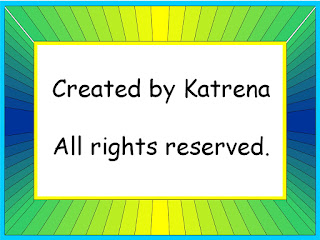Free Math Flash Cards by Katrena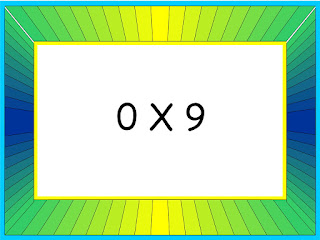0 X 90 X 9 = 0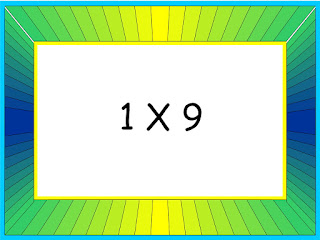1 X 91 X 9 = 92 X 92 X 9 = 183 X 93 X 9 = 27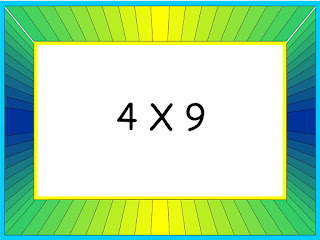4 X 94 X 9 = 365 X 95 X 9 = 45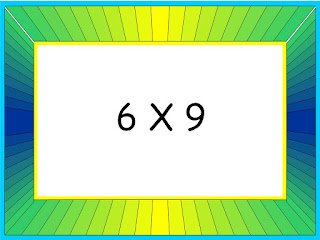6 X 96 X 9 = 54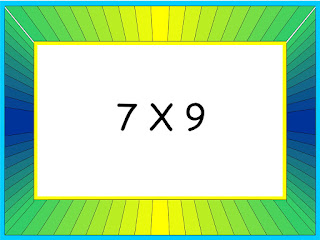7 X 97 X 9 = 63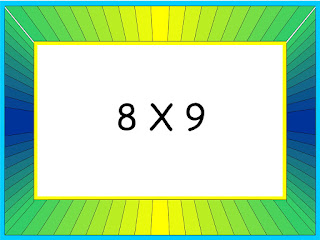8 X 98 X 9 = 729 X 9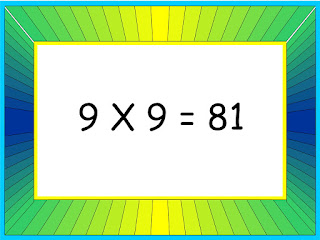9 X 9 = 8110 X 9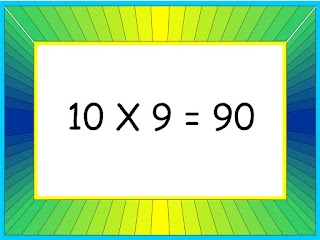10 X 9 = 90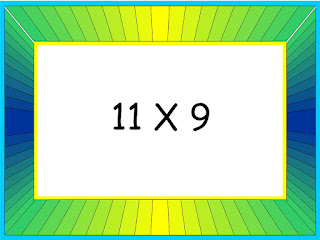11 X 9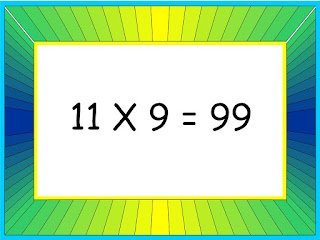11 X 9 = 9912 X 912 X 9 = 108Free Math Practice Flash Cards at Student Survive 2 Thrive